## In probability theory and statistics, the geometric distribution is either of two discrete probability distributions: (1) The probability distribution of the number x of Bernoulli trials needed to get one success or (2) The probability distribution of the number y = x − 1 of failures before the first success,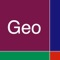# Geometric Distribution

by Donald Schaefer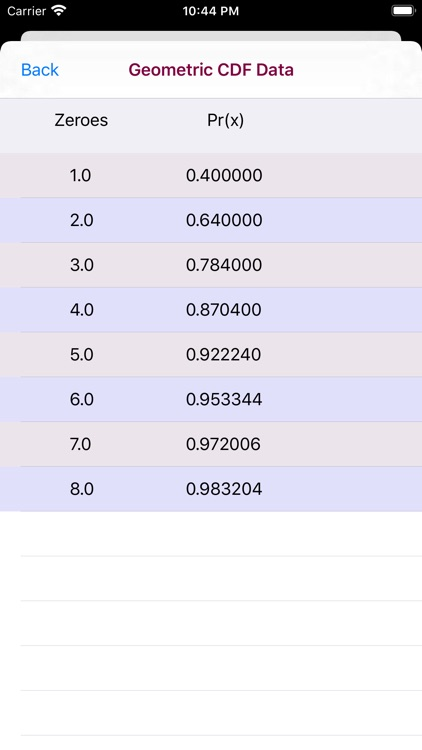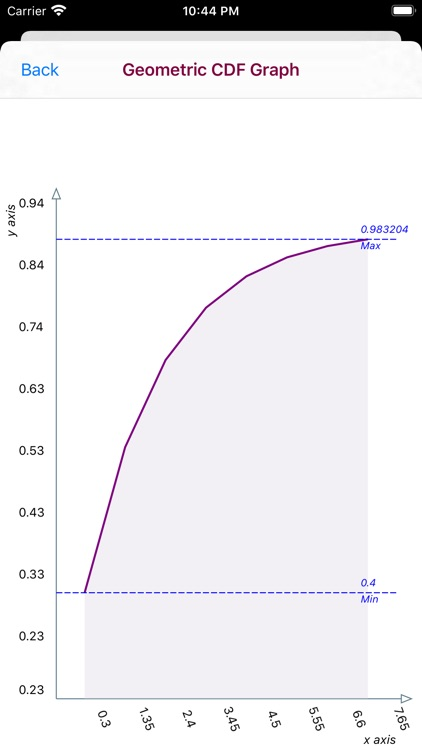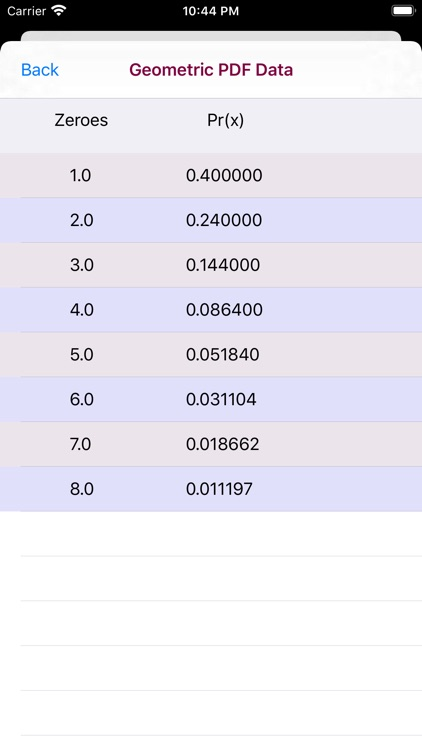Geometric Distribution Offers Apple Watch App
Check the details on WatchAware

In probability theory and statistics, the geometric distribution is either of two discrete probability distributions: (1) The probability distribution of the number x of Bernoulli trials needed to get one success or (2) The probability distribution of the number y = x − 1 of failures before the first success,### App Details

Version
1.5
Rating
NA
Size
1Mb
Genre
Education Productivity
Last updated
September 24, 2021
Release date
February 12, 2016

### App Screenshots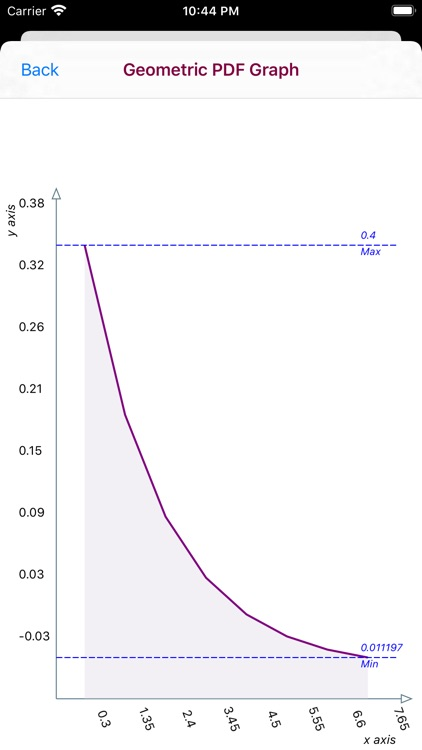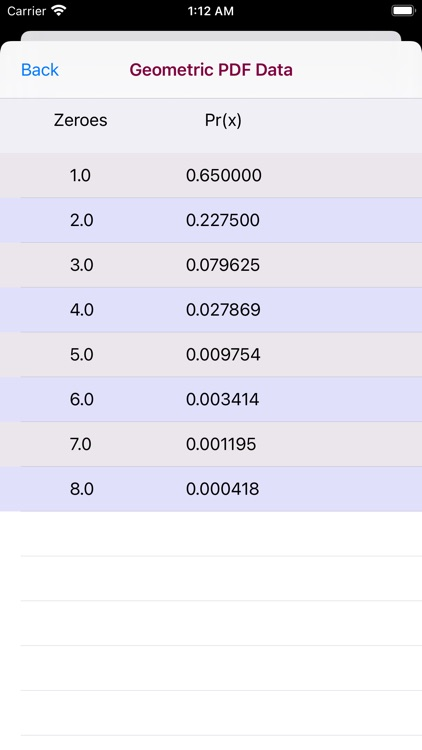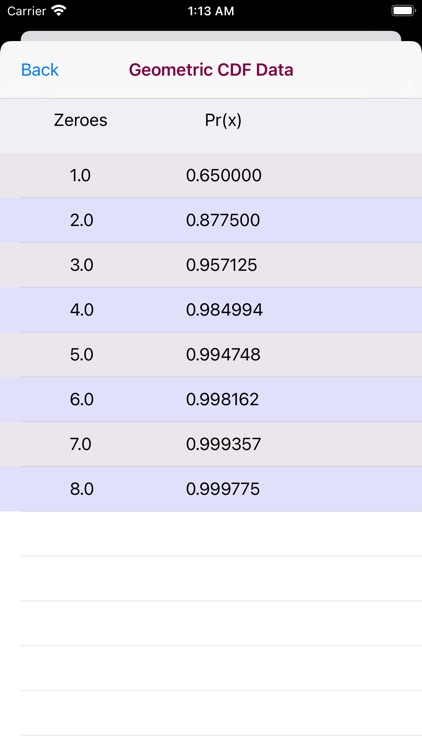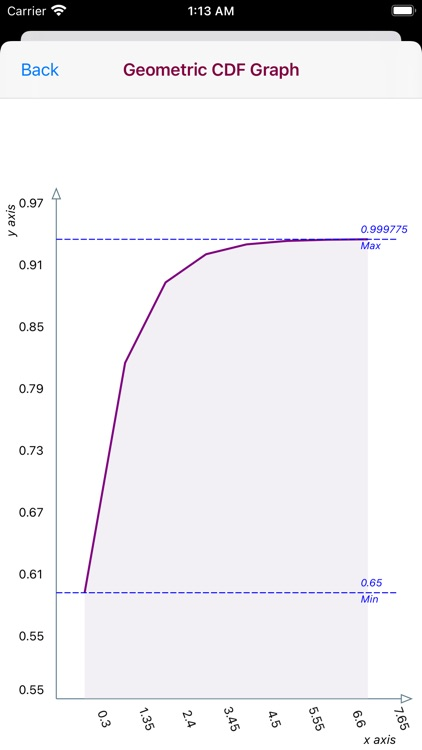### App Store Description

In probability theory and statistics, the geometric distribution is either of two discrete probability distributions: (1) The probability distribution of the number x of Bernoulli trials needed to get one success or (2) The probability distribution of the number y = x − 1 of failures before the first success,

A random variable (x) which can assume of two values (0,1) is said to have a Bernoulli Distribution if there is a constant p: 0 < p < 1, such that Pr[ x = 0 ] = 1 - p.

The Geometric Distribution app provides for the data entry of two variables: Number of Zeroes and a Probability Parameter. The range for the Number of Zeroes variable is 1.0 - 8.0 and the range of the Probability Parameter is 0.01 - 0.99.

The Geometric Distribution app produces a PDF/CDF Graph and PDF/CDF Data Tables The Number of Zeroes and Probability Parameter variables selected are used to compute the PDF/CDF data.

The PDF and CDF graphs are touch interactive graphs for computed (x/Pr(x) paired values. The graphs hav a touch feature whereby upon the touch a slidable vertical line appears. Upon movement of the line a paired (x,Pr(x) values appear relative to the line position on the graph curve.

The horizontal x-axis displays computed (x) values. The vertical y-axis plots a range of Pr(X) values.

Disclaimer:
AppAdvice does not own this application and only provides images and links contained in the iTunes Search API, to help our users find the best apps to download. If you are the developer of this app and would like your information removed, please send a request to [email protected] and your information will be removed.